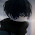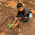## Friction (MCQs)

CBSE Class 8 - Science

Q1: The force of friction which opposes the surfaces just to slide one over the other, is called:

(A) Limiting friction.
(B) Rolling friction
(C) Sliding friction
(D) All of these.

Q2: What is the most common way to reduce friction?

(A) Lubrication.
(B) Warning signs.
(C) Eliminate the motion.
(D) None of these.

Q3: Do you want to increase or decrease friction when riding a bicycle?

(A) Increase.
(B) Both.
(C) Decrease.
(D) None of these.

Q4: Why is it harder to start to slide a heavy box across the floor than to keep it sliding?

(A) Static friction is greater than kinetic friction.
(B) Kinetic friction is greater than the static friction.
(C) Sliding friction is less than rolling friction.
(D) None.

Q5: Friction force is:

(A) Contact force.
(B) Non-Contact force.
(C) Muscular force.
(D) None of these.

Q6: Friction can be reduced by

(A) Polishing.
(B) Oiling.
(C) By use the ball bearing.
(D) All of these.

Q7: Which force among the following is a contact force:
(A) Magnetic.
(B) Electrostatic.
(C) Gravitational.
(D) Frictional.

Q8: Rocket has a special streamlined body in order to:

(A) Increase friction.
(B) Reduce friction.
(C) Make them attractive.
(D) None of these.

Q9: Burning of the meteor in the atmosphere is due to:

(A) Electrostatic force.
(B) Magnetic force.
(C) Frictional force.
(D) Gravitational force.

Q10: Fluid friction do not depend on:
(A) Speed of object.
(B) Shape of object.
(C) Nature of the fluid.
(D) Speed of the fluid.

Q11: When an object moves through a liquid, it experiences

(A) Static friction.
(B) Sliding friction.
(C) Fluid friction.
(D) Rolling friction.1.2.3.4.